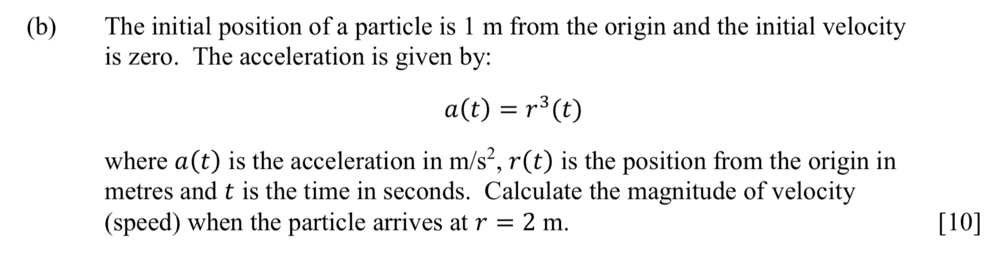# Calculate the magnitude of the velocity at point from a given equation, a(t)

Homework Statement:
Calculate the magnitude of the velocity at point from a given equation a(t)
Relevant Equations:
a(t) = r^3(t)These questions keep appearing in my past-papers and yet at no point in the course notes can I find a worked solution. Unfortunately I'm not sure where to start. My initial thoughts were regarding getting an equation using known values but w.r.t. to t and integrating for v, but like I said I have nothing to go off apart from a final answer. Any help would be greatly appreciated.

The solution for the problem included is v = 2.74m/s

I'm unsure how to get expressions for v(t) and r(t) in usable forms. I feel like once I have those expressions the remainder is relatively simple. Although I am also unsure how to treat the r^2(t) when integrating - or will this issue disappear after getting the correct expressions?

collinsmark
Homework Helper
Gold Member
Hello @emarie,

Welcome to PF!View attachment 242471

These questions keep appearing in my past-papers and yet at no point in the course notes can I find a worked solution. Unfortunately I'm not sure where to start. My initial thoughts were regarding getting an equation using known values but w.r.t. to t and integrating for v, but like I said I have nothing to go off apart from a final answer. Any help would be greatly appreciated.

The solution for the problem included is v = 2.74m/s
This is a pretty neat problem!But there are some tricks to it.

First foremost, the problem should have specified that everything is one dimensional. I.e, the acceleration is always in the same direction, which is also the same direction as the velocity and the displacement. Everything has only one, single direction. It might have made things more intuitive if the position was represented by "$x(t)$" instead of "$r(t)$." Anyway, just treat everything as a single dimension and you'll be fine.

To solve this problem you can use separation of variables together with integration.

Acceleration is the time derivative of velocity. $a = \frac{dv}{dt}$

With that, can you re-write the equation such that everything is in terms of $dv, dt,$ and $r(t)$? If so, you're almost there.

But there's still one more trick. You can't just go integrating $r^3(t) \ dt$ all willy-nilly. You don't know what the relationship is between $r(t)$ and $t$, so you'll have to do one more little step before integration.

You do know what the relationship is between $r(t)$ and $r$.
$r(t) = r.$
Wouldn't it be nice if you could integrate $r^3 \ dr$ instead? That's a pretty straight-forward integration. What do you have to multiply both sides of the equation by to make this happen?•berkeman
Hello @emarie,

Welcome to PF!This is a pretty neat problem!But there are some tricks to it.

First foremost, the problem should have specified that everything is one dimensional. I.e, the acceleration is always in the same direction, which is also the same direction as the velocity and the displacement. Everything has only one, single direction. It might have made things more intuitive if the position was represented by "$x(t)$" instead of "$r(t)$." Anyway, just treat everything as a single dimension and you'll be fine.

To solve this problem you can use separation of variables together with integration.

Acceleration is the time derivative of velocity. $a = \frac{dv}{dt}$

With that, can you re-write the equation such that everything is in terms of $dv, dt,$ and $r(t)$? If so, you're almost there.

But there's still one more trick. You can't just go integrating $r^3(t) \ dt$ all willy-nilly. You don't know what the relationship is between $r(t)$ and $t$, so you'll have to do one more little step before integration.

You do know what the relationship is between $r(t)$ and $r$.
$r(t) = r.$
Wouldn't it be nice if you could integrate $r^3 \ dr$ instead? That's a pretty straight-forward integration. What do you have to multiply both sides of the equation by to make this happen?Thank you for the help! I agree that x(t) makes more sense so that was definitely throwing me off! I have managed to find the answer by integrating the LHS wrt to r between the start and end positions and the RHS wrt to v between the start and end velocities! Thanks once again•collinsmark and berkeman